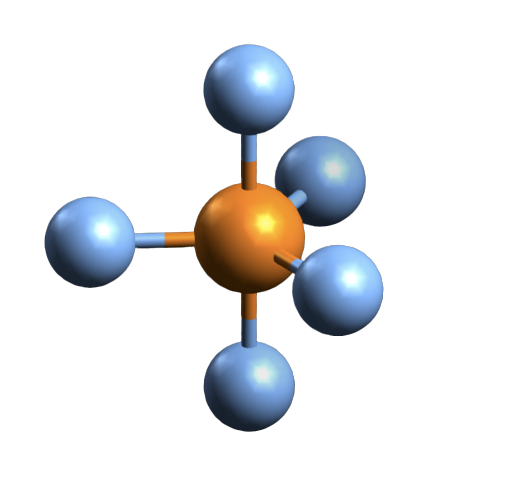Lewis Structure

# PBr5 Molecular Geometry, Lewis structure, Shape, Bond Angle, And More

Phosphorus pentabromide written as PBr5 in the chemistry equations is a reactive yellow solid. The compound has one molecule of Phosphorus and five Bromine molecules. Bromine is a halogen from Group 17 of the periodic table. Halogens are highly reactive and electronegative molecules. In the solid-state, the compound exists as PBr4+ Br- and in the gaseous state, it dissociates completely to PBr3 and Br2. It is one of those unique compounds that have a different chemical formula in varying states of matter. The compound is primarily used to convert carboxylic acids to acyl bromides.

PBr5 is highly corrosive and decomposes above 100 degree Celsius to give phosphorus tribromide and a Bromine molecule. It is vital to know the Lewis structure of a given compound to understand the molecular structure of the compound and its geometry.

Contents

## Lewis Structure of PBr5Studying the Lewis structure of a given compound helps in understanding other chemical properties of the compound such as hybridisation, polarity, etc easily. The lewis structure is defined as the pictorial representation of the electron participating in bond formation to form the compound. This representation helps to understand the basic structure of the compound. It also helps in knowing the type of charges present on the compound.

The electrons that participate in forming the bond are known as the bonding pair of electrons. Whereas the electrons that do not constitute any bonds are known as non-bonding electrons or lone electrons. The straight lines represent the bonds in the compound, and the dots represent the lone pair of electrons. All these bonding, as well as non-bonding electrons, are known as Valence electrons. The lewis structure follows the octet rule that states that for a molecule to be stable, which states that there should be eight electrons in the outer shell of the atom for a molecule to be stable.

Here in this compound, there is one molecule of Phosphorus having five valence electrons and five molecules of Bromine having seven valence electrons each.

So the total number of valence electrons is,

No. of valence electrons for Phosphorus + No. of valence electrons for Bromine

= 5 + 7*5

= 5 + 35

= 40 valence electrons total for PBr5.

Here the molecule of Phosphorus in the centre that bonds with the rest five molecules of Bromine. As the P molecule has five valence electrons, all of these electrons from the bond with one valence electron of each Bromine molecule. So there are no lone pairs of electrons on the central atom. But there are still three pairs of lone electron for each Bromine molecule. So the total number of lone electrons is 30 now.

## Hybridization of PBr5

In chemistry, the term hybridization refers to combining two or more orbitals with different energies in a compound to give a hybrid orbital. It is easy to understand the hybridization of the molecule after knowing its Lewis structure.

PBr5 has Phosphorous as the central atom has eight electrons in its outer shell after forming the bond with neighbouring halogen atoms. As the Phosphorus forms bonds with five Bromine molecules, all the electrons enter in different orbitals of the shell. The first valence electron of the halogen occupies the s orbital; next three valence electrons go in px, py and pz orbital. The last valence electron occupies the dx orbital. As one electron goes to s orbital, three occupy the p orbital, and the last one enters the d orbitals of the central atom, the hybridization of Pbr5 is sp3d.

## Molecular Geometry of PBr5In many cases, the lewis structure of the compound helps in understanding the molecular geometry of the compound. But this is an exceptional compound that requires VSEPR theory to understand its geometry. VSEPR stands for Valence Shell Electron Pair Repulsion theory that takes the steric number of the central atom and the valence electrons into consideration to know the molecular geometry.

Here the steric number for the central Phosphorus atom is 5. As the central atom is bonded with five bromine atoms, the coordination number is also 5. The repulsion between these five pairs of valence electrons can be reduced by distributing the electrons across space. Due to this reason, the PBr5 atom shows a trigonal bipyramid geometry.

## Bond Angle of PBr5

As we now know the hybridization and molecular geometry of the PBr5 molecule, it is easy to measure the bond angle. There are five pairs of bonded electrons, out of which three lie on the equatorial plane. These pairs lie along the equator of the molecule. Rest two pairs lie perpendicular to the equatorial axis known as the axial pairs. The angle between the three pairs lying on the central position is 120 degrees, and the angle between the axial and equatorial position is 90 degrees.

## Polarity of PBr5The molecular polarity of any compound depends on its geometry. In PBr5 molecule the valence electron pairs are arranged symmetrically. Due to its trigonal bipyramidal geometry, each bond is symmetrically opposed to other ones. As a result of this geometry and arrangement of electrons, the net dipole moment of the molecule is zero.  Hence there is no polarity observed in the compound and PBr5 is thus nonpolar.

### Concluding Remarks

To conclude all the properties of the molecule PBr5, it can be said that the molecule has 40 valence electrons out of which there are 15 lone pairs of electrons. The hybridization of the molecule is sp3d, and according to VSEPR theory, the compound has a trigonal bipyramidal geometry. It is a nonpolar compound as the dipole moment is nullified due to the symmetric arrangement of the lone pairs and bonded pairs of electrons.

### Janice Powell

I am interested in sharing articles related to Geometry of Molecules. It represents forms of Chemical equation with the help of structured atoms.

Close